# TSA ARMA Spectrum VI

LabVIEW 2014 Advanced Signal Processing Toolkit Help

Edition Date: June 2014

Part Number: 372656C-01

»View Product InfoDownload Help (Windows Only)

Owning Palette: Correlation and Spectral Analysis VIs

Computes the single-sided power spectral density (PSD) of a univariate time series based on autoregressive-moving average (ARMA) modeling. The PSD computed by this VI is exempt from window effects and has a better frequency resolution than the result from using the TSA Periodogram VI. This VI also matches the valleys in the spectrum better than the TSA AR Spectrum VI. Wire data to the Xt input to determine the polymorphic instance to use or manually select the instance.

Use the pull-down menu to select an instance of this VI.

 Select an instance TSA ARMA Spectrum (Waveform)TSA ARMA Spectrum (Array)

## TSA ARMA Spectrum (Waveform)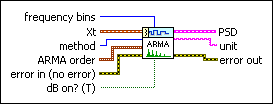frequency bins specifies the number of frequency bins for which this VI computes the single-sided power spectral density PSD. The length of the single-sided PSD is (frequency bins/2+1). The default is 1024.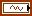Xt specifies the univariate time series.method specifies the method to use in estimating the autoregressive-moving average model.

 0 Yule-Walker (default)—Computes the AR coefficients and the MA coefficients separately with the extended Yule-Walker function based on the auto-correlation matrix. 1 High order AR—Computes the AR coefficients and the MA coefficients with a high-order AR model. 2 Polynomial—Computes the AR coefficients and the MA coefficients by solving the model-coefficient polynomial division.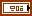ARMA order specifies the order of the autoregressive-moving average model.AR specifies the AR order of the ARMA model. The value of AR must be greater than 0. The default is 4.MA specifies the MA order of the ARMA model. The value of MA must be greater than 0. The default is 1.error in describes error conditions that occur before this node runs. This input provides standard error in functionality.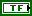dB on? specifies whether this VI returns the PSD in decibels or in a linear scale. If dB on? is TRUE, this VI returns the PSD in decibels. If dB on? is FALSE, this VI returns the PSD in a linear scale. The default is TRUE.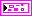PSD returns information about the single-sided power spectral density (PSD).f0 returns the lower boundary, in hertz, of the frequency range.df returns the frequency increment, in hertz.S(f) returns the magnitude of the PSD at each frequency. The value of dB on? determines the unit of measurement for this parameter.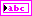unit returns the engineering unit of the PSD. You can specify an engineering unit for a time series by using the TSA Scale to EU VI.error out contains error information. This output provides standard error out functionality.

## TSA ARMA Spectrum (Array)sampling rate specifies the sampling rate, in hertz, of the univariate time series Xt. The default is 1.frequency bins specifies the number of frequency bins for which this VI computes the single-sided power spectral density PSD. The length of the single-sided PSD is (frequency bins/2+1). The default is 1024.Xt specifies the univariate time series.method specifies the method to use in estimating the autoregressive-moving average model.

 0 Yule-Walker (default)—Computes the AR coefficients and the MA coefficients separately with the extended Yule-Walker function based on the auto-correlation matrix. 1 High order AR—Computes the AR coefficients and the MA coefficients with a high-order AR model. 2 Polynomial—Computes the AR coefficients and the MA coefficients by solving the model-coefficient polynomial division.ARMA order specifies the order of the autoregressive-moving average model.AR specifies the AR order of the ARMA model. The value of AR must be greater than 0. The default is 4.MA specifies the MA order of the ARMA model. The value of MA must be greater than 0. The default is 1.error in describes error conditions that occur before this node runs. This input provides standard error in functionality.dB on? specifies whether this VI returns the PSD in decibels or in a linear scale. If dB on? is TRUE, this VI returns the PSD in decibels. If dB on? is FALSE, this VI returns the PSD in a linear scale. The default is TRUE.PSD returns information about the single-sided power spectral density (PSD).f0 returns the lower boundary, in hertz, of the frequency range.df returns the frequency increment, in hertz.S(f) returns the magnitude of the PSD at each frequency. The value of dB on? determines the unit of measurement for this parameter.error out contains error information. This output provides standard error out functionality.

## TSA ARMA Spectrum Details

This VI computes the PSD of a univariate time series based on autoregressive-moving average (ARMA) modeling according to the following equation: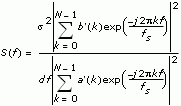where S(f) is the PSD of the time series, df is the frequency interval, which is computed as fs/N. N is the number of frequency bins, fs is the sampling rate, and s2 is the estimated noise series of the ARMA model of the time series. a is an array that contains the AR coefficients of the ARMA(m, n) model, and a=[1, a1, a2,…, am]. b is another array that contains the MA coefficients of the ARMA(m, n) model, and b=[1, b1, b2,…, bn]. Before computing the PSD, this VI wraps a, b to N-point series a', b'.

The minimum length requirement for the input time series differs for each method you use:

• Yule-Walker method: minimum length ≥ AR order + MA order
• High-Order AR method: minimum length ≥ 5 × MA order
• Polynomial method: minimum length ≥ 5 × (AR order + MA order)

## Example

Refer to the Power Spectral Density Estimation VI in the labview\examples\Time Series Analysis\TSAGettingStarted directory for an example of using the TSA ARMA Spectrum VI.